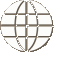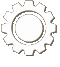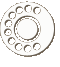COURSE Material
Course picture not available.
Foundations and Pre-Calculus Mathematics 10

Foundations and Pre-Calculus of Mathematics 10 is divided into seven units. It meets the required outcomes outlined by Saskatchewan Learning, and is a prerequisite for Foundations of Mathematics 20, and Pre-Calculus 20. Students will require a TI-83 or TI-84 calculator in order to complete this course. This workbook series was developed for use by schools using an individualized, mastery-based learning system, and therefore comes with score keys, tests, and test keys. No additional text is necessary.

Unit 1: Measurement

At the end of unit one, the student should be able to:

• use proportional reasoning and conversion factors to convert measurements in the SI metric and Imperial measurement systems
• solve problems involving the surface areas of right pyramids and right cones
• solve problems involving the volumes of right pyramids and right cones
• solve problems involving the surface area and volume of a sphere

Unit 2: Trigonometry

At the end of unit two, the student should be able to:

• develop and apply the sine, cosine, and tangent ratios to determine angle measures
• use the sine, cosine, and tangent ratios to determine side lengths
• use sine, cosine, and tangent ratios to solve problems modelled by right triangles

Unit 3: Factors and Products

At the end of unit three, the student should be able to:

• determine prime factors, greatest common factors, and least common multiples of whole numbers
• apply algebraic strategies to multiply binomials and to factor trinomials
• apply algebraic strategies to multiply polynomials
• factor special polynomials (trinomial squares and difference of squares)

Unit 4: Radicals and Exponents

At the end of unit four, the student should be able to:

• express radical expressions in varying forms and as decimal expressions
• identify and order irrational numbers
• express entire radicals as mixed radicals, and vice versa
• express fractional exponents as radicals, and vice versa
• relate negative exponents to reciprocals
• apply the exponent laws to simplify expressions

Unit 5: Relations and Functions

At the end of unit five, the student should be able to:

• define relation, ordered pair, abscissa, and ordinate
• graph ordered pairs in the Cartesian co-ordinate plane, and to graph real-world relations in the Cartesian coordinate plane
• read information from a graph, interpolate information between two given ordered pairs and extrapolate information by extending the line of the graph
• identify, graph, and interpret examples of linear functions describing real-world situations and distinguish between linear and non-linear functions
• determine if a relation is a function by employing the vertical line test
• graph a linear function using a table of values
• solve equations in two variables, given the domain of one of the variables
• determine if an ordered pair is a solution to the linear equation
• observe that the solution set for an equation consists of all the ordered pairs that lie on the graph of the equation and that parentheses have to be used when substituting in for variables
• construct scatter plots from real-world data using the graphic calculator
• interpret and critically analyze these scatter plots

Unit 6: Equations of Linear Functions

At the end of unit six, the student will be able to:

• calculate the slope of a line graphically; algebraically; from the equation
• determine the slope of horizontal and vertical lines
• identify lines that have positive, negative, zero, and undefined slopes
• determine the slope of parallel and perpendicular lines
• graph a linear equation in two variables using:
• x and y intercepts
• the slope and an ordered pair
• the slope and y - intercept
• write linear equations in slope-intercept form and in standard form
• write the equation of a line when given:
• slope and y - intercept
• slope and one point on the line
• the graph of the line
• two points on the line

Unit 7: Systems of Linear Equations

At the end of unit seven, the student will be able to:

• develop systems of linear equations
• solve a system of linear equations graphically
• solve a system of linear equations, using a graphic calculator
• solve a system of linear equations by substitution
• solve a system of linear equations by elimination
COURSE MaterialsSAICS has developed Christian curriculum (where none other was available) to match Saskatchewan Learning objectives for high school courses.

View Our Course Listing
ORDERING CoursesFind out more information about ordering courses that have been developed by SAICS including score keys and associated textbooks.

Ordering Information